2022: SklogWiki celebrates 15 years on-line

# 1-dimensional Ising model

The 1-dimensional Ising model is an Ising model that consists of a system with$N$ spins in a row. The energy of the system is given by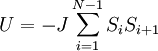$U = -J \sum_{i=1}^{N-1} S_{i} S_{i+1}$,

where each variable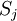$S_j$ can be either -1 or +1.

The partition function of the system will be: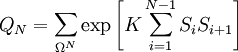$Q_N = \sum_{\Omega^N } \exp \left[ K \sum_{i=1}^{N-1} S_i S_{i+1} \right]$,

where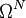$\Omega^N$ represents the possible configuration of the N spins of the system, and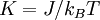$K = J/k_B T$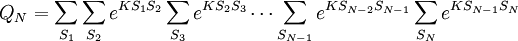$Q_{N} = \sum_{S_1} \sum_{S_2} e^{K S_1S_2} \sum_{S_3} e^{K S_2 S_3} \cdots \sum_{S_{N-1}} e^{K S_{N-2} S_{N-1}}\sum_{S_{N}} e^{K S_{N-1} S_{N} }$

Performing the sum of the possible values of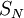$S_{N}$ we get: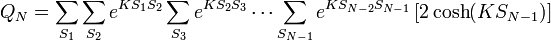$Q_{N} = \sum_{S_1} \sum_{S_2} e^{K S_1S_2} \sum_{S_3} e^{K S_2 S_3} \cdots \sum_{S_{N-1}} e^{K S_{N-2} S_{N-1}} \left[ 2 \cosh ( K S_{N-1} ) \right]$

Taking into account that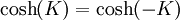$\cosh(K) = \cosh(-K)$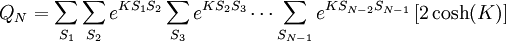$Q_{N} = \sum_{S_1} \sum_{S_2} e^{K S_1S_2} \sum_{S_3} e^{K S_2 S_3} \cdots \sum_{S_{N-1}} e^{K S_{N-2} S_{N-1}} \left[ 2 \cosh ( K ) \right]$

Therefore: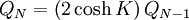$Q_{N} = \left( 2 \cosh K \right) Q_{N-1}$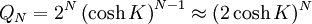$Q_N = 2^{N} \left( \cosh K \right)^{N-1} \approx ( 2 \cosh K )^N$

The Helmholtz energy function in the thermodynamic limit will be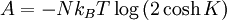$A = - N k_B T \log \left( 2 \cosh K \right)$